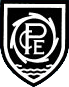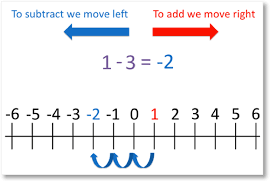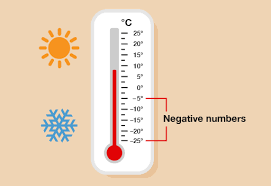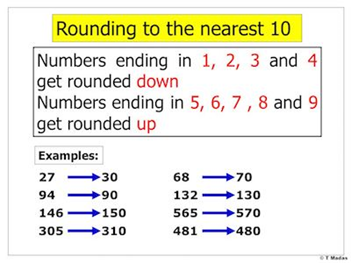# Learning  »  Class Pages  »  Year 4  »  Class Work

Week 7
Mrs Young (Monday - Tuesday)

This week the children have been learning about negative numbers and where you would use them.Week 6
Mrs Young (Monday - Tuesday)
The children have been continuing to learn about rounding, and have been rouding numbers to the nearest 100 and 1000. They have been completing a range of activities linked to this.Mrs Doughty (Wednesday - Friday)
This week we have looked at different strategies for subtracting, including column subtraction and using a numberline. We looked at how we 'exchange' tens (or hundreds, or thousands) if we do not have 'enough' in one column to subtract the digit. We used lots of images to help the children understand this:We also looked at a different strategy for when we need to subtract from multiples of 100 or 1000. In this case, it can be easier to use a numberline to count UP to the larger number. We can call this finding the difference. We used bar models to support understanding, and also looked at lots of different 'real life' problems where we could use this skill. Again - we realied how important it is that we know our number bonds to 10!Week 5
Mrs Young (Monday - Tuesday)
This week we have been looking at finding 1000 more and less than a given number. We have also looked at rounding any number to the nearest ten.Mrs Doughty (Wednesday -Friday)
This week we have looked at formal methods of column addition with up to 4 digits. We have discussed the importance of place value and lining up digits correctly:We have looked at what is happening when we have more than 9 in any column, and how we must remember to add in the additional ten, hundred or thousand in the correct collumn.We have also looked at solving problems with collumn addition, and looking at how to calculate missing numbers:Week 4
Mrs Young (Monday -Tuesday)
We have been looking at different ways of representing 3 and 4 digit numbers using numberals, words and pictures. For example 345 = 300 + 40 + 5 = 34 tens and 5 ones = three hundred and forty five. We have also looked at numberlines and ordering numbers up to 10,000.

Mrs Doughty (Wednesday - Friday)
This week we have looked at adding multiples of 10 and 100 to 3 and 4 digit numbers. We have used lots of models and images to help the children understand that  10 ones = 1 ten, 10 tens = 1 hundred, 10 hundreds = 1 thousand. We have discussed which digits of numbers will change when we add or subtract numbers which 'bridge' ten.Week 3
Mrs Young (Monday -Tuesday)

The children have been comparing and ordering numbers up to 1000 and beyond. Alongside this, they have also been showing their understanding of how they recognise the value of each digit in a 3 and 4 digit number.

Mrs Doughty (Wednesday - Friday)
This week we have used the same ideas as last week to look at how we can subtract ones from 2, 3 and 4 digit numbers. We have also looked at patterns in numbers, for example that when we add any number that has 7 in the ones, to any other number that has 5 in the ones column, the answer will always have 2 in the ones:
237 + 5 = 242                547 + 25 = 582                 2547  + 5 = 2552

.This week we also recapped 3x table multiplication facts to support children with their TT Rockstars at home.

Week 2
Mrs Young (Monday -Tuesday)

We will be focusing on 'Place value' in Maths and checking that the children can recognise the place value of each digit in a 3 and 4 digit number. The children will be completing a range of activities to show their confidence of using numbers and representing them in different ways.

Mrs Doughty (Wednesday - Friday)
This week the children have looked at mental strategies for adding  to 2 and 3 digit numbers that 'cross tens'. We looked at how part-whole models can help us to do this, along with number bonds to 10:Pool-in-Wharfedale CE Primary School Arthington Lane, Pool-in-Wharfedale, Otley, LS21 1LG